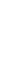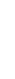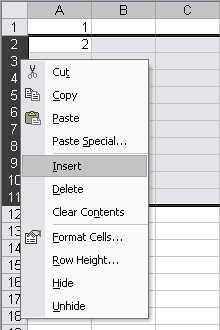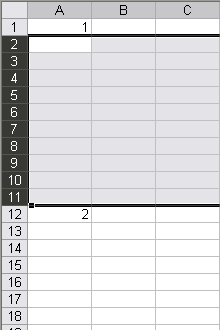# Excel tip: Inserting multiple rows at once: the Excel way and the easy way

Date: 13 november 2006

## Inserting multiple rows "the Excel way"

If you want to insert multiple rows at once the standard procedure is to first select multiple rows and then use the "Insert" command.
For example if you want to insert 10 rows between A1 and A2, you first have to select rows 2:11 and then insert the rows.## Inserting multiple rows "the easy way"

The above solution works for small amount of rows. However if you need to insert for example 800 rows this is not a workable solution. Therefore I have written the following macro which lets you choose the amount of rows to insert.
Sub sbInsertMultipleRows()
' Insert multiple rows at once. Rows are inserted above the
' currently selected row/cell
Dim lNewRows                           As Long
Dim lCurrentRow                        As Long

' detect if current selection is a row or cell
If UCase(TypeName(Selection)) <> "RANGE" Then
MsgBox "Please select an initial row or cell to insert rows above.", _
vbInformation
Exit Sub
End If

' Let user choose an amount of rows to insert:
lNewRows = Application.InputBox("Number of rows to insert", _
"Insert multiple rows", 1, , , , , 1)

' Cancel if amount is 0 or user choose to cancel:
If lNewRows <= 0 Then Exit Sub

' Insert the rows:
Rows(Selection.Cells(1).Row & ":" & _
Selection.Cells(1).Row + lNewRows - 1).Insert shift:=xlDown

End Sub

« back

Printer friendly page
© 1999-2019 - - - - The Netherlands